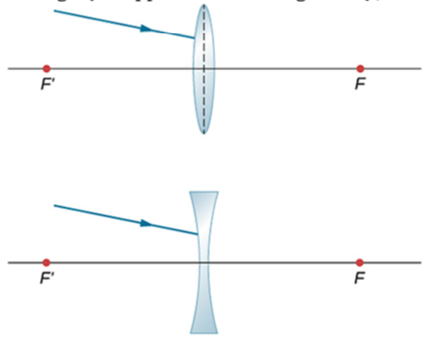×
Get Full Access to University Physics, Volume 3 - 17 Edition - Chapter 2 - Problem 107
Get Full Access to University Physics, Volume 3 - 17 Edition - Chapter 2 - Problem 107

×ISBN: 9781938168185 2032

## Solution for problem 107 Chapter 2

University Physics, Volume 3 | 17th Edition

• Textbook Solutions
• 2901 Step-by-step solutions solved by professors and subject experts
• Get 24/7 help from StudySoup virtual teaching assistantsUniversity Physics, Volume 3 | 17th Edition

4 5 1 327 Reviews
15
1
Problem 107

Microscopes and Telescopes

Unless otherwise stated, the lens-to-retina distance is 2.00 cm.

Trace rays to find which way the given ray will emerge after refraction through the thin lens in the following figure. Assume thin-lens approximation. (Hint: Pick a point P on the given ray in each case. Treat that point as an object. Now, find its image Q. Use the rule: All rays on the other side of the lens will either go through Q or appear to be coming from Q.)Step-by-Step Solution:
Step 1 of 3

Physics Projectile Motion ­New equa2ions R(θ ) = Vo sinθ2)/g h= Vo^2 sin^2( θ ) / 2θ) 2 t= Vi ± √ −2gd+Vo / g ­Describe motion of object in 2 dimensions ­Ball is in free fall vertically and moves at a constant speed horizontally ­Force is in opposite direction to ball’s velocity vector and is proportional to the velocity at relatively low speeds ­Keep it simple by considering motion close to the surface of the earth for the time being ­Neglect air resistance to make it simpler ­An object falling is in free fall vertically and moves at a constant speed horizontally ­ What happens when we add air resistance Adds a new force on the ball ­The force is in the opposite direction to the ball’s velocity vector and is proportional to the velocity at relatively low speeds ­Need calculus to sort out the resulting motion ­Lowers the angle for maximum range The trick to maximum range is just to keep the object off the ground for as long as possible. ­This allows the horizontal motion to be a maximum since x = vxt ­Make range longer by going higher for your starting point ­Make range longer by having more velocity Communications Satellites ­Farther out you go, the bigger the circumference of the orbit ­It takes longer for the trip ­Also, gravity weakens by inverse square law the farther out you go ­Make the distance so that it takes 24 hours for the orbit ­Satellite is stationary in the sky!!! Kepler’s Laws ­Each planet moves in an elliptical orbit with the sun at one focus of the ellipse. ­The line from the sun t

Step 2 of 3

Step 3 of 3

##### ISBN: 9781938168185

Since the solution to 107 from 2 chapter was answered, more than 202 students have viewed the full step-by-step answer. University Physics, Volume 3 was written by Aimee Notetaker and is associated to the ISBN: 9781938168185. The full step-by-step solution to problem: 107 from chapter: 2 was answered by Aimee Notetaker, our top Physics solution expert on 03/18/22, 10:28AM. This full solution covers the following key subjects: . This expansive textbook survival guide covers 11 chapters, and 1216 solutions. This textbook survival guide was created for the textbook: University Physics, Volume 3 , edition: 17. The answer to “?Microscopes and TelescopesUnless otherwise stated, the lens-to-retina distance is 2.00 cm.Trace rays to find which way the given ray will emerge after refraction through the thin lens in the following figure. Assume thin-lens approximation. (Hint: Pick a point P on the given ray in each case. Treat that point as an object. Now, find its image Q. Use the rule: All rays on the other side of the lens will either go through Q or appear to be coming from Q.)” is broken down into a number of easy to follow steps, and 81 words.

## Discover and learn what students are asking

Calculus: Early Transcendental Functions : Basic Differentiation Rules and Rates of Change
?How Do You See It? Use the graph of f to answer each question. To print an enlarged copy of the graph, go to MathGraphs.com. (a) Betwee

Unlock Textbook Solution